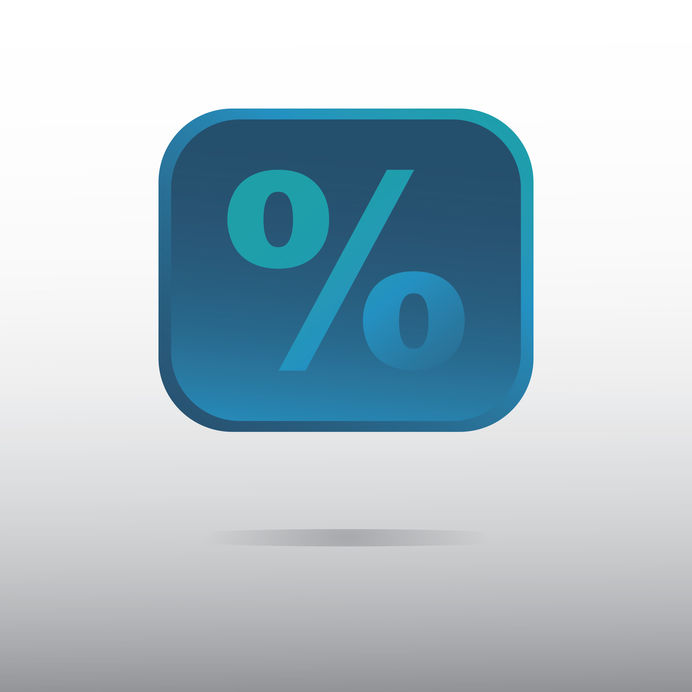# The Blog of Mathedu Academy

### A content for the curious of the mathematics in the real world… and beyond, including video tutorials.# Add a percentage is a Multiplication

Add a percentage, say 5% is not making an addition. It is a multiplication by (1+5/100). You may also multiply by 5, divide by 100, and then add the result to the original amount.

For instance, the VAT of 20% multiplies the taxes free amount by 1.2, or else adds 20% of the taxes free amount to itself.

That's why the VAT amount of 20% is NOT 20% of the price, as the price is the taxes included amount. To obtain the VAT amount from the price, you must first DIVIDE the price by 1.2, to obtain the taxes free price, and then take 20% of that taxes free amount.

You may also multiply the price by 20/1.2, that is about 16.67, and then divide it by 100: the VAT is about 16.67% of the final price, which is far less than 20%.

Another example is a saving account with an interest rate of 2%, were you put $10,000 for a long duration. If you do not retrieve the interests after 1 year (2% of$10,000 is $200), after 2 years, you will have more than 2 times$200 of interests. Indeed, you will have a capital $10,200 multiplied by (1+2/100)=1.02, that is$10,404.

The interests after 2 years are $404, that is$4 more than 2 times $200. It is 0.04% of$10,000.
And if you are very patient and wait 8 years, you will har a capital of $10,000 multiplied by 1.02 to the power 8 (multiplied by itself 8 times), that is$11,716.60. The interests are $1,716.6 instead of 8 times 200,$1,600. You get $116,60 more than you may have thought, that is 1.166% of$10,000!

This is great, isn't it?

But beware, for the interests of a loan, it is the contrary: it costs more than you may think: it is recommended to ask a simulation to the bank!

And if you wish to learn more on the percentages manipulation, there is a